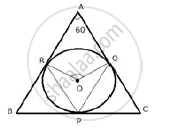Share

# In a Triangle Abc, the Incircle (Centre O) Touches Bc, Ca and Ab at Points P, Q and R Respectively. Calculate : (I) ∠Qor - Mathematics

Course

#### Question

In a triangle ABC, the incircle (centre O) touches BC, CA and AB at points P, Q and R respectively. Calculate : (i) ∠QOR

#### SolutionThe incircle touches the sides of the triangle ABC and
OP ⊥  BC,OQ ⊥ AC,OR ⊥ AB

∠ORA = 90°  ,∠OQA = 90°  ,∠A = 60°
∠QOR = 360°  - (90°  + 90° + 60° )

∠QOR = 360° -  240°
∠QOR = 120°

Is there an error in this question or solution?
In a Triangle Abc, the Incircle (Centre O) Touches Bc, Ca and Ab at Points P, Q and R Respectively. Calculate : (I) ∠Qor Concept: Tangent Properties - If a Line Touches a Circle and from the Point of Contact, a Chord is Drawn, the Angles Between the Tangent and the Chord Are Respectively Equal to the Angles in the Corresponding Alternate Segments.